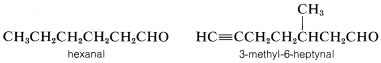# 7.4: Aldehydes

$$\newcommand{\vecs}{\overset { \rightharpoonup} {\mathbf{#1}} }$$ $$\newcommand{\vecd}{\overset{-\!-\!\rightharpoonup}{\vphantom{a}\smash {#1}}}$$$$\newcommand{\id}{\mathrm{id}}$$ $$\newcommand{\Span}{\mathrm{span}}$$ $$\newcommand{\kernel}{\mathrm{null}\,}$$ $$\newcommand{\range}{\mathrm{range}\,}$$ $$\newcommand{\RealPart}{\mathrm{Re}}$$ $$\newcommand{\ImaginaryPart}{\mathrm{Im}}$$ $$\newcommand{\Argument}{\mathrm{Arg}}$$ $$\newcommand{\norm}{\| #1 \|}$$ $$\newcommand{\inner}{\langle #1, #2 \rangle}$$ $$\newcommand{\Span}{\mathrm{span}}$$ $$\newcommand{\id}{\mathrm{id}}$$ $$\newcommand{\Span}{\mathrm{span}}$$ $$\newcommand{\kernel}{\mathrm{null}\,}$$ $$\newcommand{\range}{\mathrm{range}\,}$$ $$\newcommand{\RealPart}{\mathrm{Re}}$$ $$\newcommand{\ImaginaryPart}{\mathrm{Im}}$$ $$\newcommand{\Argument}{\mathrm{Arg}}$$ $$\newcommand{\norm}{\| #1 \|}$$ $$\newcommand{\inner}{\langle #1, #2 \rangle}$$ $$\newcommand{\Span}{\mathrm{span}}$$$$\newcommand{\AA}{\unicode[.8,0]{x212B}}$$

1. The suffix -al is appended to the name of the hydrocarbon corresponding to the longest carbon chain that includes the aldehyde carbon. Remember that alkane $$+$$ -al becomes alkanal with the e omitted, and because the al function is necessarily at $$C1$$, the -1- is redundant and is omitted:Dialdehydes are named as -dials. Thus $$OHCCH_2CH_2CH_2CH_2CHO$$ is hexanedial.

2. The simplest aldehyde is methanal, $$HCHO$$, which is familiarly known as formaldehyde. However, when aldehydes are named as derivatives of methanal, they usually are called carbaldehydes, and the suffix "carbaldehyde" refers to the $$-CHO$$ group. This system is used where the hydrocarbon group is not a chain, but a ring, and the $$CHO$$ group can be thought of as a one-carbon chain:3. When the $$-CHO$$ group is a substituent on the parent chain or ring and it ranks below another functional group, it properly is designated by the prefix methanoyl. However, the prefix formyl also is used:(The naming of acids will be discussed more in detail in Section 7-6.)

4. Trivial names are used for many simple aldehydes, some of which are shown below in parentheses: